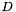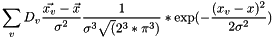Gromacs  2020.1
gmx::DensityFittingForce Class Reference

#include <gromacs/math/densityfittingforce.h>

## Description

Manages evaluation of density-fitting forces for particles that were spread with a kernel.

The density fitting force is the inner product of the derivative of the density-density goodness-of-fit function at all density values and the gradient of the spreading kernel used to simulate the density.

For a discrete Gauss transform spreading kernel and density derivativeit is the Gauss transform of a single coordinate, weighted by a distance vector and density derivative at each lattice point## Classes

class  Impl
Private implementation class for DensityFittingForce. More...

## Public Member Functions

Construct density fitting force evaluator. More...

DensityFittingForce (const DensityFittingForce &other)
Copy constructor.

DensityFittingForceoperator= (const DensityFittingForce &other)
Copy assignment.

DensityFittingForce (DensityFittingForce &&other) noexcept
Move constructor.

DensityFittingForceoperator= (DensityFittingForce &&other) noexcept
Move assignment.

RVec evaluateForce (const GaussianSpreadKernelParameters::PositionAndAmplitude &localParameters, basic_mdspan< const float, dynamicExtents3D > densityDerivative)
Evaluate density-fitting force between a reference density and the density generated by a kernel. More...

## Constructor & Destructor Documentation

 gmx::DensityFittingForce::DensityFittingForce ( const GaussianSpreadKernelParameters::Shape & kernelShapeParameters )

Construct density fitting force evaluator.

Parameters
 [in] kernelShapeParameters the global parameters of the density spreading kernel

## Member Function Documentation

 RVec gmx::DensityFittingForce::evaluateForce ( const GaussianSpreadKernelParameters::PositionAndAmplitude & localParameters, basic_mdspan< const float, dynamicExtents3D > densityDerivative )

Evaluate density-fitting force between a reference density and the density generated by a kernel.

Implements the equation in the class description.

Parameters
 [in] localParameters local parameters of the spreading kernel [in] densityDerivative the spatial derivative of the similarity measure between the reference density and a density that was generated using the spreading kernel
Returns
the force that increases the measure of the goodness of fit used to calculate the density derivative

The documentation for this class was generated from the following files: